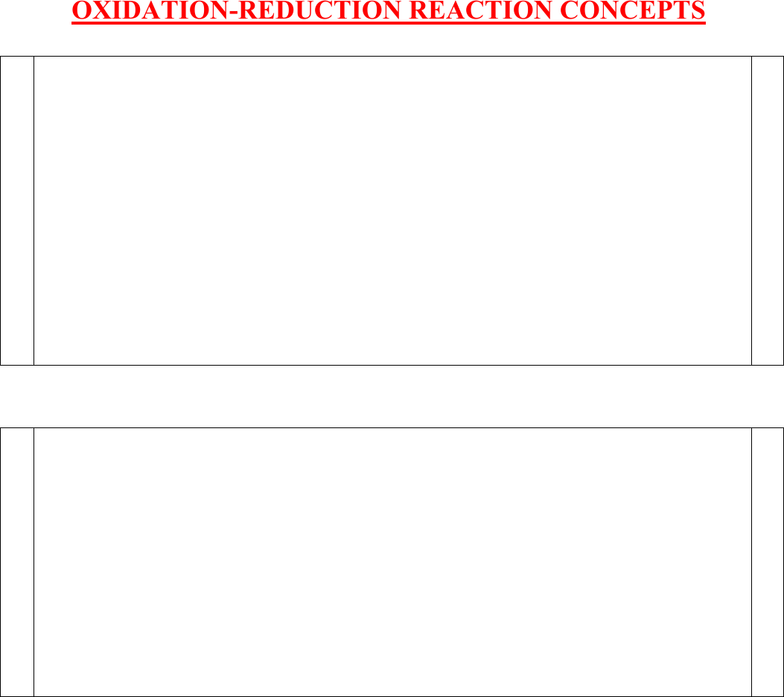Study Guides (380,000)
US (220,000)
Rutgers (3,000)
3:40 (100)
Final

01:160:161 Chapter Notes - Chapter 20: Electrochemistry, Joule, Lithium ChlorideExam

Department
Chemistry
Course Code
01:160:161
Professor
Asbed Vassilia
Study Guide
Final

This preview shows pages 1-3. to view the full 26 pages of the document.1
B&O CHAPTER 20
ELECTROCHEMISTRY
Problems to prepare students for Chem 162 final exam.
OXIDATION-REDUCTION REACTION CONCEPTS
OXIDATION-REDUCTION REACTION CALCULATIONS
GALVANIC CELLS & REDUCTION-POTENTIAL
CONCEPTS
REDUCTION POTENTIAL CALCULATIONS
FREE ENERGY, EQUILIBRIA AND CELL POTENTIAL
CALCULATIONS/NERNST EQUATION
ELECTROLYSIS
ELECTRIC WORK/AMPERES
BATTERIES AND CORROSION
E. Tavss, PhD
4/10/13

Only pages 1-3 are available for preview. Some parts have been intentionally blurred.2
OXIDATION-REDUCTION REACTION CONCEPTS
19 Chem 161-2011 Final exam
B&O CHAPTER 9 – CHEMICAL REACTIONS IN AQUEOUS SOLUTIONS
Oxidation-Reduction reaction concepts (H&P Chapter 18)
Consider the following balanced redox reaction:
2Cr3+(aq) + 4OH-(aq) +3MnO2(s) Æ 2CrO42-(aq) + 2H2O(l) + 3Mn2+(aq)
Which of the following is true?
A. Cr3+ is the oxidizing agent
B. The element Mn is reduced
C. OH- is reduced to H2O
D. OH- is oxidized to H2O
E. The reaction occurs in acidic solution.
31 Chem 161-2011 Final exam
B&O CHAPTER 9 – CHEMICAL REACTIONS IN AQUEOUS SOLUTIONS
Oxidation-Reduction reaction concepts (H&P Chapter 18)
Which one of the following is a redox reaction?
A. 2Na(g) + Cl2(g) 2NaCl(s)
B. Ba2+(aq) + SO42-(aq) BaSO4(s)
C. K2Cr2O7(aq) + 2KOH(aq) 2K2CrO4(aq) + H2O(l)
D. Na2CO3(s) + 2HCl(aq) 2NaCl(aq) + CO2(g) + H2O(l)
E. H2O(l) H+(aq) + OH-(aq)
Chem 161-2011 Exam III
Burdge and Overby Chapter 9 – Chemical Reactions in Aqueous Solutions
Oxidation-Reduction Reaction Concepts, H&P Ch 18
4. In the reaction
Zn(s) + H2SO4(aq) ZnSO4(aq) + H2(g)
which element, if any, is oxidized?
a) hydrogen
b) sulfur
c) oxygen

Only pages 1-3 are available for preview. Some parts have been intentionally blurred.3
d) zinc
e) none of them
Chem 161-2011 Exam III
Burdge and Overby Chapter 9 – Chemical Reactions in Aqueous Solutions
Oxidation-Reduction Reaction Concepts, H&P Ch 18
5. Which one of the following reactions is an oxidation-reduction reaction?
a) LiCl(aq) + AgCH3CO2(aq) AgCl(aq) + LiCH3CO2(aq)
b) HF(aq) + KOH(aq) H2O(l) + KF(aq)
c) 3Mg(s) + 2FeCl3(aq) 3 MgCl2(aq) + 2Fe(s)
d) Al3+(aq) + PO43-(aq) AlPO4(s)
e) NaCl(aq)+ AgNO3(aq) NaNO3(aq)+ AgCl(s)
.
Chem 161-2011 Exam III
Burdge and Overby Chapter 9 – Chemical Reactions in Aqueous Solutions
Oxidation-Reduction Reaction Concepts, H&P Ch 18
22. The oxidation number of chromium in Cr2O72- is
a) 0
b) 7/2
c) 6
d) 7
e) 8
13 Chem 162-2011 Final exam
Chapter 18 – Electrochemistry
Oxidation-reduction reaction concepts
What is the coefficient of H+ when the following skeletal reaction is balanced in acid with the
lowest possible set of integer coefficients?
IO3-(aq) + Tl+ +Cl- ICl2- + Tl3+
A. 6
B. 5
C. 4
D. 3
E. 2